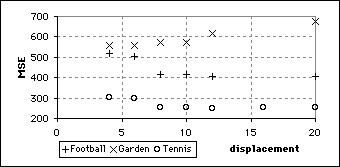## Greedy Algorithm B ResultsNumber of criteria evaluations required by Greedy Algorithm B as the maximum displacement was increasedMSE of sequences produced by coded with Greedy Algorithm B with various displacements

All three sequences were coded using Greedy Algorithm B with various maximum displacements. As with Greedy Algorithm A, the number of criteria evaluations was independent of the sequence coded. The graph above suggests that it exhibited step behaviour, but there are too few data to be sure. For the Football and Tennis sequence the MSE was at a minimum at ±12 pixels. This value is surprisingly high, and is most likely the result of this algorithm starting with a smaller initial step size. Because the initial step size is one quarter of the maximum displacement rather than one half, the algorithm can operate with larger displacements while concentrating the initial searches in the area close to the centre of the search area. Perhaps this behaviour holds lessons applicable to other algorithms, such as the TDL.
The lowest MSE for Algorithm B was lower than that for Algorithm A.
Like Greedy Algorithm A, Algorithm B had a very small operative range when coding the Garden sequence.